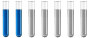Determining mass for solutions

Chemistry and homework help forum.

Organic Chemistry, Analytical Chemistry, Biochemistry, Physical Chemistry, Computational Chemistry, Theoretical Chemistry, High School Chemistry, Colledge Chemistry and University Chemistry Forum.

Share your chemistry ideas, discuss chemical problems, ask for help with scientific chemistry questions, inspire others by your chemistry vision!

Please feel free to start a scientific chemistry discussion here!

Discuss chemistry homework problems with experts!

Ask for help with chemical questions and help others with your chemistry knowledge!

Moderators: expert, ChenBeier, Xen

IneedhelpwithChem
Jr. MemberPosts: 7
Joined: Sat Nov 06, 2021 11:17 am

Determining mass for solutions

Hi everyone. I have an assignment which requires me to solve this.
"Determine the mass of KClO3 required to make a 0.5M solution. Atomic masses: K = 39, Cl =35.5, O = 16"

Can someone please tell me what steps I need to go through to get the answer, what do I need to find? I know how to calculate molar mass and such, i'm just not sure of the steps I need to take to get the answer for the question.
ChenBeier
Distinguished MemberPosts: 1004
Joined: Wed Sep 27, 2017 7:25 am
Location: Berlin, Germany

Re: Determining mass for solutions

0,5 M means 0,5 mol/l
Calculate molar Mass, this the weight for 1 mol. Calculate then for 0,5 mol. This mass put in a measured flask and fill up to 1l with water
IneedhelpwithChem
Jr. MemberPosts: 7
Joined: Sat Nov 06, 2021 11:17 am

Re: Determining mass for solutions

Molar mass of KCLO3 is 39 + 35.5 + 16(x3) = 125.5
= 125.5g/ 1 mole
this is what I have so far, what next?
ChenBeier
Distinguished MemberPosts: 1004
Joined: Wed Sep 27, 2017 7:25 am
Location: Berlin, Germany

Re: Determining mass for solutions

No
The molar mass is not correct its 122,5 g/mol, but can you read above.
There is it written how to go further.
IneedhelpwithChem
Jr. MemberPosts: 7
Joined: Sat Nov 06, 2021 11:17 am

Re: Determining mass for solutions

yes, so I have to divide the moral mass in half to get the answer for .5 mol? and then add water?? I don't understand completely
ChenBeier
Distinguished MemberPosts: 1004
Joined: Wed Sep 27, 2017 7:25 am
Location: Berlin, Germany

Re: Determining mass for solutions

Yes it is the half of this amount. This has to be dissolved in water to get 1 l.
IneedhelpwithChem
Jr. MemberPosts: 7
Joined: Sat Nov 06, 2021 11:17 am

Re: Determining mass for solutions

wow........guess I overcomplicated it, thank you so much
IneedhelpwithChem
Jr. MemberPosts: 7
Joined: Sat Nov 06, 2021 11:17 am

Re: Determining mass for solutions

Molar mass of KCLO3 is 39 + 35.5 + 16(x3) = 122.5
= 122.5g per 1 mole solution
Molar mass of KCLO3 for 0.5M solution = 122.5g divided by 2
= 61.25g per .5 mole solution
ChenBeier
Distinguished MemberPosts: 1004
Joined: Wed Sep 27, 2017 7:25 am
Location: Berlin, Germany

Re: Determining mass for solutions

IneedhelpwithChem wrote: Sat Nov 06, 2021 12:29 pm Molar mass of KCLO3 is 39 + 35.5 + 16(x3) = 122.5
= 122.5g per 1 mole solution
Molar mass of KCLO3 for 0.5M solution = 122.5g divided by 2
= 61.25g per .5 mole solution
The Molar mass is 122,5 g/mol. The mass of 0,5 mol is 61,25 g.
After dissolving it in water up to 1 l!!!! it is 0,5 mol/l